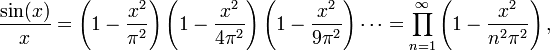## Thursday, February 12, 2009

### Math Poettary - Infinite

Check out the post here.

A brief explanation

The post mentions the sphere as a "one-point compactification" of the (complex) plane (by adding a point at infinity). The property of the sphere being compact somehow makes it a little closer to being "finite" and therefore easier to handle. But to understand more precisely what all this means you need to take a good course in Complex Analysis or Topology.

When studying complex analysis, I thought that the theorems are simpler, more beautiful, and closer to the finite case than analogous theorems in Real Analysis. I don't know whether that is due to the relationship with the sphere, but I suspect it is so.

Here is an example: You know that a polynomial p(x) with real coefficients (and a finite degree) can be written as a product of factors of the form (x-a) where a is a zero of the polynomial. (The root a is of-course a complex number). Turns out, under certain conditions, we can write a function (which can be viewed as an infinite series) as an (infinite) product of its zeros. For example, consider this formula:

Euler's Product:(The formula above taken from Wikipedia's entry on the Wallis Product.)

The formula looks nicer if you replace x by (pi)x. Then the expression on the left has zeros at +1, +2, +3, ... and -1, -2, -3, .... And on the right you get factors of the form (1-x/n)(1+x/n) which is zero for x = +n and -n.
In fact, the way we write the product is something to do with making the product "converge" (or make sense).

This formula is definitely something I will write about one day. I think I need to pick up a complex analysis book again...its been too long...and have almost forgotten the beautiful stuff I used to see everyday.